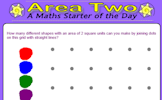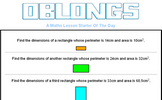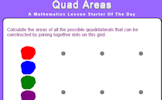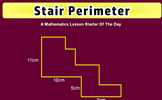# Area, Perimeter and Volume

### Term 2 starting in week 8 :: Estimated time: 2 weeks

• Shapes - same area
• Area and perimeter
• Area of a triangle
• Area of parallelogram
• What is volume? (review)
• Volume counting cubes
• Volume of a cuboid

This page should remember your ticks from one visit to the next for a period of time. It does this by using Local Storage so the information is saved only on the computer you are working on right now.

## Lesson Starters

Here are some suggestions for whole-class, projectable resources which can be used at the beginnings of each lesson in this block.

### 1st Lesson#### Area Two

How many different shapes with an area of 2 square units can you make by joining dots on this grid with straight lines?

### 2nd Lesson#### Cross Perimeter

Calculate the distance around the given shape

### 3rd Lesson#### Missing Square Puzzle

The missing square puzzle is an optical illusion used to help students reason about geometrical figures.

### 4th Lesson#### Oblongs

Find the dimensions of a rectangle given the perimeter and area.

### 5th LessonCalculate the areas of all the possible quadrilaterals that can be constructed by joining together dots on this grid.

### 6th Lesson#### Stair Perimeter

Use the information implied in the diagram to calculate the perimeter of this shape.

Some of the Starters above are to reinforce concepts learnt, others are to introduce new ideas while others are on unrelated topics designed for retrieval practice or and opportunity to develop problem-solving skills.

White Rose ResourcesEnd of block assessments provide a quick progress check at the end of each block of learning to make sure students have understood the content covered. This Scheme of Learning was produced by White Rose Maths and is used here with permission granted on 30th June 2021.For All: